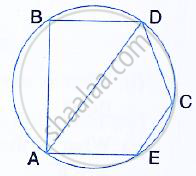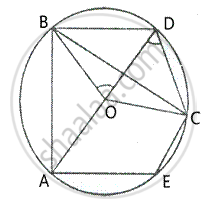Share

# In the Given Figure, Bd is a Side of a Regular Hexagon, Dc is a Side of a Regular Pentagon and Ad is a Diameter. Calculate : - Mathematics

Course

#### Question

In the given figure, BD is a side of a regular hexagon, DC is a side of a regular pentagon and AD is a diameter. Calculate :(i) ∠ADC (ii) ∠BDA, (iii) ∠ABC, (iv) ∠AEC.

#### SolutionJoin BC, BO, CO and EO
Since BD is the side of a regular hexagon,

∠BOD = = 360/6 =60°
Since DC is the side of a regular pentagon,
∠COD = 360/5 = 72°
In ∆BOD. ∠BOD = 60° and OB = OD
∴ ∠OBD = ∠ODB = 60°

(i) In ∆OCD, ∠COD = 72° and OC = OD

∴ ∠ODC = 1/2 (180° - 72°)
= 1/2 xx 108°
= 54°

(ii) ∠BDO = 60° or ∠BDA = 60°

(iii) Arc AC subtends ∠AOC at the centre and
∠ABC at the remaining part of the circle.
∴ ∠ ABC = 1 /2∠AOC
= 1/2[∠AOD - ∠COD]
= 1/2 xx (180° - 72°)
= 1/2 xx108°
= 54°

∠AEC + ∠ADC = 180°               [sum of opposite angles]
⇒ ∠AEC + 54° = 180°
⇒ ∠AEC = 180° - 54°
⇒ ∠AEC = 126°

Is there an error in this question or solution?